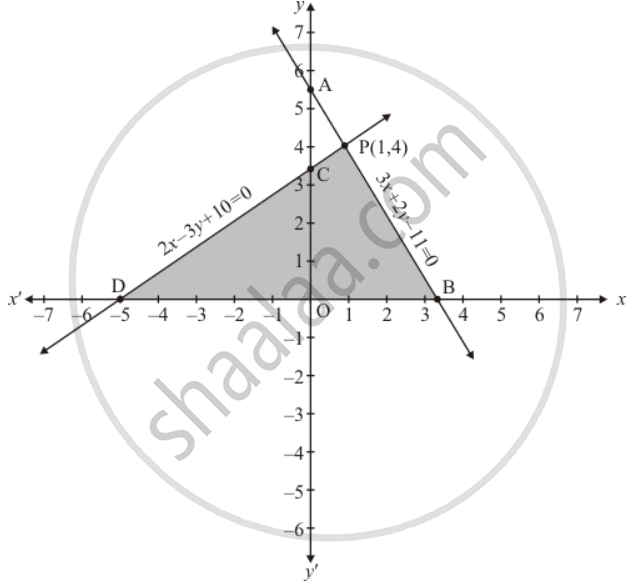Share

# Solve the Following System of Linear Equations Graphically and Shade the Region Between the Two Lines and X-axis: 3x + 2y − 11 = 0 2x − 3y + 10 = 0 - CBSE Class 10 - Mathematics

ConceptGraphical Method of Solution of a Pair of Linear Equations

#### Question

Solve the following system of linear equations graphically and shade the region between the two lines and x-axis:

3x + 2y − 11 = 0

2x − 3y + 10 = 0

#### Solution

The given equations are:

3x + 2y − 11 = 0  ....(i)

2x − 3y + 10 = 0 ....(ii)

Putting x = 0 in equation (i) we get

=> 3 xx 0 + 2y = 11

=> y = 11/2

x = 0, y = 11/2

Putting y = 0 in eqaution (i) we get

=> 3x + 2 xx 0 = 11

=> x = 11/3

x = 11/3, y = 0

Use the following table to draw the graph.

 x 0 11/3 y 11/2 0

Draw the graph by plotting the two points A(0,11/2), B(11/3, 0) from table2x - 3y + 10 = 0 ....(ii)

Putting x = 0 in equation (ii) we get

=> 2 xx 0 - 3y = - 10

=> y = 10/3

x = 0, y = 10/3

Putting y = 0 in equation (ii) we get

=> 2x - 3 xx 0 = -10

=> x = - 5

x = -5, y = 0

Use the following table to draw the graph.

 x 0 -5 y 10/3 0

Draw the graph by plotting the two points C(0,10/3), D(-5,0) from table.

The two lines intersect at P(1,4). The area enclosed by the lines represented by the given equations and the coordinates x−axis and shaded the area in the graph.

Hence, x = 1 and y = 4 and is the solution.

Is there an error in this question or solution?

#### APPEARS IN

Solution Solve the Following System of Linear Equations Graphically and Shade the Region Between the Two Lines and X-axis: 3x + 2y − 11 = 0 2x − 3y + 10 = 0 Concept: Graphical Method of Solution of a Pair of Linear Equations.
S